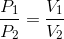# MCAT Physical : Other Gas Principles

## Example Questions

### Example Question #31 : Gases

An ideal gas with a volume of one liter at STP is placed into a piston chamber that can freely change volume. The volume is measured as the temperature is lowered. The gas will achieve its minimal volume at __________.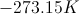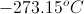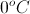its boiling point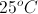its boiling point

Explanation:

The best answer is the compound's boiling point. At this point, it ceases obeying the gas laws and becomes a liquid.

If it were possible to remain in a gaseous state to zero Kelvin (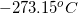), then this would be the correct answer. As the gas changes to a liquid, it becomes effectively resistant to further compression and will occupy the same volume through any further decrease in temperature.

### Example Question #2 : Other Gas Principles

Diffusion can be defined as the net transfer of molecules down a gradient of differing concentrations. This is a passive and spontaneous process and relies on the random movement of molecules and Brownian motion. Diffusion is an important biological process, especially in the respiratory system where oxygen diffuses from alveoli, the basic unit of lung mechanics, to red blood cells in the capillaries.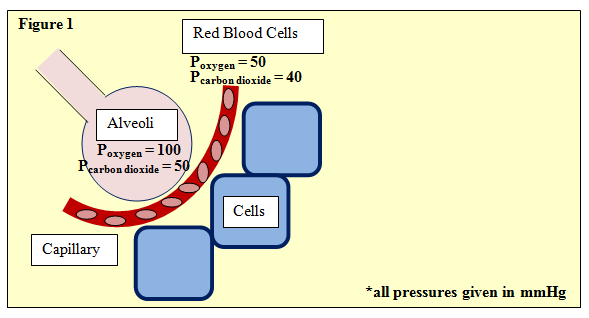Figure 1 depicts this process, showing an alveoli separated from neighboring cells by a capillary with red blood cells. The partial pressures of oxygen and carbon dioxide are given. One such equation used in determining gas exchange is Fick's law, given by:

ΔV = (Area/Thickness) · Dgas · (P1 – P2)

Where ΔV is flow rate and area and thickness refer to the permeable membrane through which the gas passes, in this case, the wall of the avlveoli. P1 and P2 refer to the partial pressures upstream and downstream, respectively. Further, Dgas­, the diffusion constant of the gas, is defined as:

Dgas = Solubility / (Molecular Weight)^(1/2)

At an alveoli-capillary diffusion equilibrium, which of the following is true?

There is a 1:1 exchange of oxygen.

There is a 5:1 exchange of carbon dioxide.

There is a 2:1 exchange of oxygen.

There is a 4:1 exchange of carbon dioxide.

There is a 1:1 exchange of oxygen.

Explanation:

This is also a straightforward question that can be answered from little to no information from the passage. The point of emphasis here is equilibrium. At a diffusion equilibrium there is no net change, and thus, a one-to-one oxygen molecule exchange fits the bill.

### Example Question #1 : Other Gas Principles

Gas X has a density of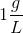and gas Y has a density of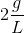. Equal volumes of the two gases are sealed in a container, which is then punctured by a small pin. Which gas diffuses faster out of the container?

Gas Y because it has a higher molar mass than gas X

Gas X because it has a higher molar mass than gas Y

Gas X because it has a lower molar mass than gas Y

Gas Y because it has a lower molar mass than gas X

Gas X because it has a lower molar mass than gas Y

Explanation:

To solve this question, we can use Graham's law of effusion: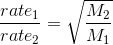Graham's law demonstrates that the rate of effusion is inversely proportional to the square root of the molar mass. Essentially, lighter gases will effuse more quickly than heavier gases.

Given the densities and equal volumes of the two gases, we see that the gas with the greater density will account for more molar mass. The volume of gas Y weighs twice as much as the volume of gas X. Based on this information, we can conclude that gas X diffuses faster because it has a lower molar mass.

### Example Question #2 : Other Gas Principles

Which equation best describes Boyle's law?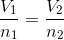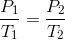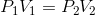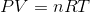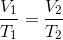Explanation:

Boyle's law describes the inverse relationship between a change and pressure and a change in volume, while a sample of gas is kept at constant temperature. This relationship can be mathematically written as:or